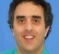## raised-cosine filter paramiters.Started by 5 years ago3 replieslatest reply 5 years ago875 views

I have 228000 samples of 1 second BPSK signal (with noise) which is shaped with raised-cosine pulse, rollof factor = 1. Signal data rate is 1187.5 symbols/s. I tried to obtain receive filter in matlab using rcosdesign function and then use filtfilt in order to convolve it with my signal.

I was sure that value: sps (which I assume means SamplesPerSymbol) will be 228000/1187.5 = 192 (samples per second divided by symbols per second), but output of filtfilt is 100% bad.

matlab code: https://pastebin.com/jvM1b63E

plot ('before filter' is multiplie by 90):

[ - ]I don't know about that specific Matlab function, but I woulod assume 'sps' to stand for samples-per-second and thus 228000...

[ - ]The MATLAB on-line documentation for the rcosdesign function states that sps means Samples Per Second:

### sps — Samples per symbolpositive integer scalar

Number of samples per symbol (oversampling factor), specified as a positive integer scalar.

Data Types: double | single

[ - ]b = rcosdesign(beta,span,sps,shape) returns a square-root raised cosine filter when you set shape to 'sqrt' and a normal raised cosine FIR filter when you set shape to 'normal'.